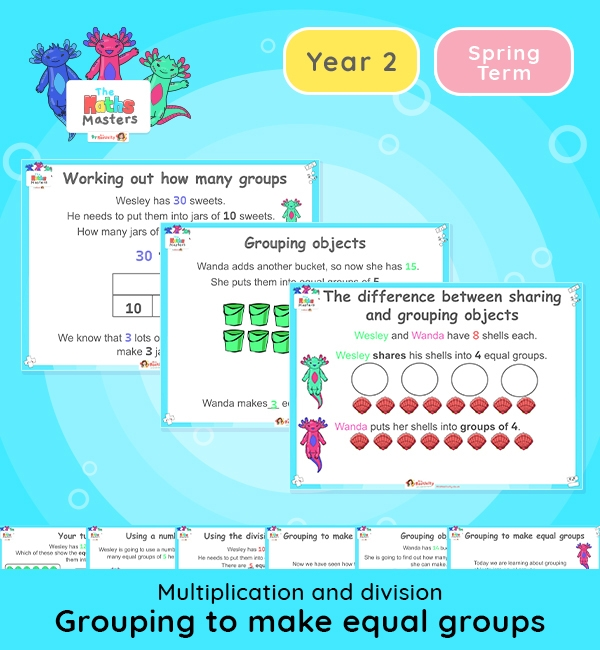# Year 2 | Grouping to Make Equal Groups Lesson Presentation## White Rose Year 2 multiplication and division lesson plans

Aligned with the White Rose Maths scheme of work, this Year 2 | Grouping to Make Equal Groups Lesson Presentation is fully editable, and is designed for the Year 2 maths curriculum covering the following maths objectives for the autumn term:

Topic: Multiplication and division

This lesson practises grouping numbers of objects to make equal groups. It may be preferable to spread the learning over two sessions.

The first half of the presentation practises grouping objects into equal groups and recaps skills taught in the summer term of Year 1. The second half moves onto using division calculations and the link between grouping objects and multiplication/repeated addition.

White Rose Maths Small Steps: Make equal groups – grouping.

NC Links: Recall and use multiplication and division facts for the 2, 5, and 10 times tables. *Calculate mathematical statements for multiplication and division within the multiplication tables and write them using the multiplication, division and equals signs. *Solve problems involving multiplication and division using materials, arrays, repeated addition, mental methods and multiplication and division facts including problems in context. *Count in steps of 2, 3, 5, and 10.

TAF Statements: Working At  – Recall and use multiplication and division facts for 2, 5, and 10 and use them to solve simple problems.

Greater Depth – Recall and use multiplication and division facts for 2, 5, and 10 and make deductions outside known multiplication facts. *Use reasoning about number and relationships to solve more complex problems and explain their thinking.

Ready-to-progress criteria:  2MD-2 Relate grouping problems where the number of groups is unknown to multiplication equation with a missing factor and two division equations (quotitive division)

Year 1 Conceptual prerequisites: Count in multiples of 2, 5, and 10 to find how many groups of 2, 5 or 10 there are in a particular quantity, set in everyday contexts.

## Recently Viewed